# Difference between revisions of "Ethane"This article is a 'stub' page, it has no, or next to no, content. It is here at the moment to help form part of the structure of SklogWiki. If you add sufficient material to this article then please remove the {{Stub-general}} template from this page.

  200 lightgrey Ethane.pdb  Ethane

Ethane.

## Models

The NERD parameters are:

 Molecule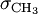$\sigma_{\mathrm {CH}_3}$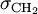$\sigma_{\mathrm {CH}_2}$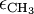$\epsilon_{\mathrm {CH}_3}$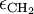$\epsilon_{\mathrm {CH}_2}$ ethane 3.825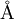$\mathrm{\AA}$ 3.93$\mathrm{\AA}$ 100.6 K

## Critical properties

The pressure, temperature and density at the critical point have been calculated for a virial equation of state using the TraPPE-UA force field, and are given in Table I of .

## Virial coefficients

The virial coefficients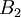$B_2$-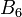$B_6$ as a function of temperature for the TraPPE force field have been tabulated by Schultz and Kofke .